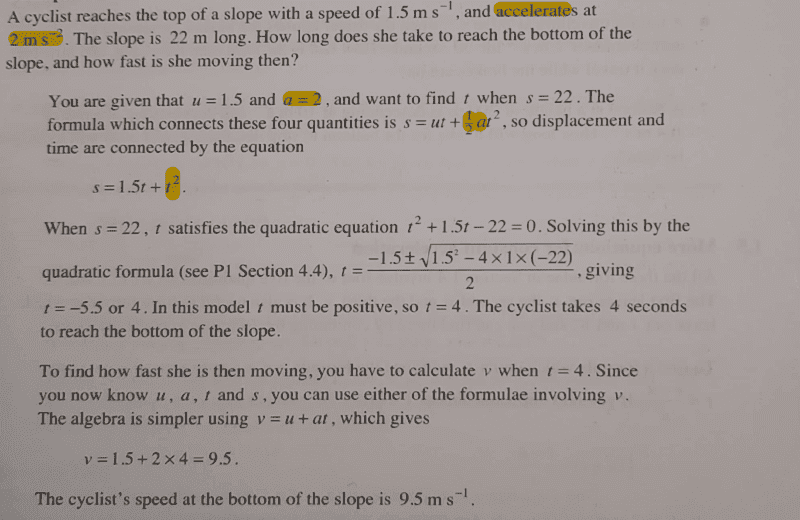# Find the time taken to travel from top of slope to base - Mechanics

• chwala
In summary, the cyclist's initial velocity is 1.5 m/s and his acceleration is 2 m/s2 when he reaches the top of the slope.f

#### chwala

Gold Member
Homework Statement
Kindly see attached
Relevant Equations
##s##=##ut##+##\frac {1}{2}####at^2##
Now this is a textbook example with solution.I understand working to solution...my only reservation is on how they used acceleration. The cyclist, i understand was traveling at a constant acceleration of ##2## ##m/s^2## before reaching the top part of the slope.
Now, if he is descending, which outrightly is deceleration or rather retardation, then are we assuming that he is traveling at the same constant rate of acceleration?

ok, i think i get it now...When going down the slope we are now going to have our initial velocity being ##u=1.5####m/s##...
i had to imagine/visualize a cyclist in motion going up a slope then change of direction to negative slope (still in motion)...then it is clear that the initial velocity will be ##1.5##m/s^2##...cheers guys...Last edited:
I understand working to solution...my only reservation is on how they used acceleration. The cyclist, i understand was traveling at a constant acceleration of ##2## ##m/s^2## before reaching the top part of the slope.
That's not how I read it. I interpreted it to mean that the cyclist's acceleration is 2 m/s2 starting at the top. However, this is irrelevant because the position and speed at the top are both known, so it doesn't matter what happened before.

Now, if he is descending, which outrightly is deceleration or rather retardation, then are we assuming that he is traveling at the same constant rate of acceleration?
Why deceleration? The cyclist is going faster going down the hill, which is usually what happens! The speed is to be considered here with respect to the ground, not along two Cartesian coordinates.

ok, i think i get it now...When going down the slope we are now going to have our initial velocity being ##u=1.5####m/s##...
i had to imagine/visualize a cyclist in motion going up a slope then change of direction to negative slope (still in motion)...then it is clear that the initial acceleration will be ##2####m/s^2##...cheers guys...See above.

That's not how I read it. I interpreted it to mean that the cyclist's acceleration is 2 m/s2 starting at the top. However, this is irrelevant because the position and speed at the top are both known, so it doesn't matter what happened before.

Why deceleration? The cyclist is going faster going down the hill, which is usually what happens! The speed is to be considered here with respect to the ground, not along two Cartesian coordinates.

See above.
Thanks. Noted with regards...